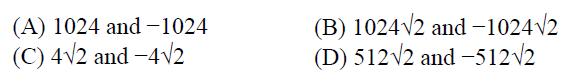Open In App

# GATE | GATE CS 2012 | Question 11

Let A be the 2 × 2 matrix with elements a11 = a12 = a21 = +1 and a22 = −1. Then the eigenvalues of the matrix A19 are(A) A
(B) B
(C) C
(D) D

Explanation:

```A =  1    1
1   -1

A2 = 2   0
0   2

A4 = A2 X A2
A4 = 4   0
0   4

A8 = 16   0
0    16

A16 = 256   0
0    256

A18 = A16 X A2
A18 = 512   0
0    512

A19 = 512   512
512  -512

Applying Characteristic polynomial

512-lambda   512
512       -(512+lambda)  =   0

-(512-lambda)(512+lambda) - 512 x 512 = 0

lambda2 = 2 x 5122 ```

Alternative solution:

```det(A) = -2.
det(A^19) = (det(A))^19 = -2^19 = lambda1*lambda2.
The only viable option is D. ```

Thanks to Matan Mandelbrod for suggesting this solution.